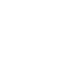# SAT Question of the Day (ACT Bonus!): Mar. 3, 2014

If you are reading this in an email you received from me, do not click the link to sat.collegeboard.org below. Use the link to my website that is farther down on the email.

http://sat.collegeboard.org/practice/sat-question-of-the-day?questionId=20140303&oq=1 (This link takes you to today’s question. If you use my archive, you will see the question related to my SAT explanation for that date.)

The answer is D.  Always begin by asking yourself, what is the topic of the sentence and then predict words for the blanks that are consistent with that topic.  This sentence tells us the author refutes (that is, disagrees with) the claim that if you express your anger, you will reduce it.  The author actually believes that is wrong and that releasing or expressing (my prediction for the first word) can increase its level or amount (my prediction for the second word).

Now here is a great strategy to use when there are two blanks.  Begin with the prediction you feel is stronger.  I happen to like my second one a little better; so, let’s start looking for a second word that means level or amount.  Only Answer D, intensity is a word that has to do with level or amount.  Looking at the first word for Answer D, I know that venting means releasing.  Perfect.  I’m all done.

What should you learn from this question?  First, when faced with two blanks, make two predictions and use one of them to eliminate as many answers as possible before considering the other blank.  This approach will save you a lot of time and sometimes, like today, you don’t even need to worry about the other word!  Second, you need to look for key words and phrases in the sentence (refuting and can actually) and you should circle or underline them.  In this case, refuting and can actually tell you there’s a change in direction and the author disagrees with the claim or statement about expressing anger.  Third, the level of difficulty of the question is often not associated with how hard the words in the answers are.  (Only 55% of students are getting this question correct but the words in the answers are not that difficult.)  It is often a key word (refute) in the sentence itself that is essential.  Finally, keep an eye out for “directional” words or as I often say, “Check the charges (positive or negative) of the words.”  The test writers like to switch directions or charges — or give you key words that tell you they stay the same.  These approaches are especially true when there are two blanks.

I wonder if the ACT folks have something new for us this morning.

Nothing new here!  This is the slope-intercept equation, y = mx + b, where m is the slope and b is the y-intercept.

The answer is B.  Recognizing the good, old slope-intercept equation answers this question.  Solving for y, the coefficient of x  is -3/5 and the constant is 2.  That means the slope is -3/5 which gives us Answer B.  But read on because it is not as important for you to get this question correct as it is to see what you can learn about the ACT and SAT tests by doing it.

Today’s question is an easy way the slope-intercept equation is tested because it is straight forward.  However, you could see a slope-intercept equation question that is in a more difficult format such as 3x = 10 – 5y  which is the same equation that is in today’s question.  Then they may ask, “Which of the following equations is parallel to this equation on the coordinate grid?”  You need to restate (Pillar II) the question as, “Which equation has the same slope?”  That is the signal that this equation is a slope-intercept and you need to solve for y  so that you have a coefficient for x.  Then find the answer that has a slope of -3/5 and you are all done.

When you are reading my blog.  Be sure that you don’t stop reading once you determine that you got the answer correct!  The biggest value in reading my blog is in what you can learn about the test.  After all, this question is not going to be on your test but what you learned about the test will certainly be helpful on test day.

I had a great group of students at Olympia High this weekend.  Now I’m looking forward to moving up the road to Apopka High on Wednesday to meet the Blue Darters.

Bob Alexander, the “SAT and ACT Wizard”